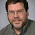### Hyperbolic Pascal triangles and other stories

A new set of mathematical abstracs. We start with the hyperbolic Pascal trianlges:Fibonacci and Pell sequences in the hyperbolic Pascal triangle
In this paper, we introduce a new generalization of Pascal's triangle. The new object is called the hyperbolic Pascal triangle since the mathematical background goes back to regular mosaics on the hyperbolic plane. We describe precisely the procedure of how to obtain a given type of hyperbolic Pascal triangle from a mosaic. Then we study certain quantitative properties such as the number, the sum, and the alternating sum of the elements of a row. Moreover, the pattern of the rows, and the appearence of some binary recurrences in a fixed hyperbolic triangle are investigated.
Hacene Belbachir, László Németh & László Szalay (2015). Hyperbolic Pascal triangles, arXiv:
A new representation for pythagorean triples:Geometric interpretation of $m$ and $n$
In this paper we introduce a formula that parameterises the Pythagorean triples as elements of two series. With respect to the standard Euclidean formula, this parameterisation does not generate the Pythagorean triples where the elements of the triple are all divisible by 2. A necessary and sufficient condition is also proposed for a Pythagorean triple obtained from this formula to be primitive.
$a = −2n + 4nm + 4m^2 − 4m+1$ $b = 2n^2 − 2n + 4nm$ $c = 2n^2 − 2n + 4nm + 4m^2 − 4m + 1$.
Anthony Overmars & Lorenzo Ntogramatzidis (2015). A new parameterisation of Pythagorean triples in terms of odd and even series, arXiv:
And in conclusion a story about the lexicographic representation of numbers:
It is proven that, contrarily to the common belief, the notion of zero is not necessary for having positional representations of numbers. Namely, for any positive integer $k$, a positional representation with the symbols for $1$, $2$, $\dots$, $k$ is given that retains all the essential properties of the usual positional representation of base $k$ (over symbols for $0$, $1$, $2$, $\dots$, $k−1$). Moreover, in this zero-free representation, a sequence of symbols identifies the number that corresponds to the order number that the sequence has in the ordering where shorter sequences precede the longer ones, and among sequences of the same length the usual lexicographic ordering of dictionaries is considered. The main properties of this lexicographic representation are proven and conversion algorithms between lexicographic and classical positional representations are given. Zero-free positional representations are relevantt in the perspective of the history of mathematics, as well as, in the perspective of emergent computation models, and of unconventional representations of genomes.
Vincenzo Manca (2015). On the lexicographic representation of numbers, arXiv:

#### 1 comment:

1.Using the digits 1,2,... in base k expansion is well-known and not new. The earliest reference I know is Salomaa's book Formal Languages (pp. 90-91) in 1973, although it is probably much older. Other references include Forslund, A logical alternative to the existing positional number system, Southwest J. Pure Appl. Math. 1 (1995), 27-29 and Boute, Zeroless positional number representation and string ordering, Amer. Math. Monthly 107 (2000), 437-444.

Markup Key:
- <b>bold</b> = bold
- <i>italic</i> = italic
- <a href="http://www.fieldofscience.com/">FoS</a> = FoS